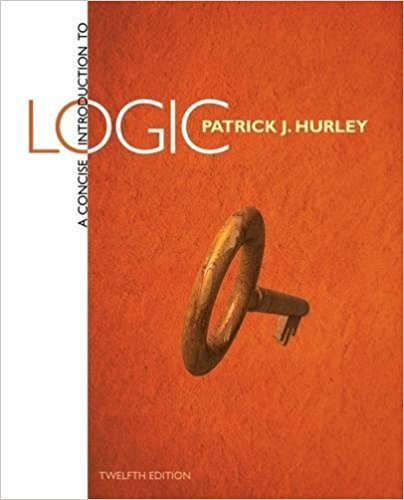×
×

# New answer: Interpret the following symbolized arguments in light of the eight argumentISBN: 9781285196541 340

## Solution for problem 9 Chapter 6.6

A Concise Introduction to Logic | 12th Edition

• Textbook Solutions
• 2901 Step-by-step solutions solved by professors and subject experts
• Get 24/7 help from StudySoup virtual teaching assistantsA Concise Introduction to Logic | 12th Edition

4 5 1 316 Reviews
13
1
Problem 9

Interpret the following symbolized arguments in light of the eight argument forms presented in this section. In some cases a symbolized argument must be rewritten using commutativity or double negation before it becomes an instance of one of these forms. Those not having a named form are invalid.B L G B G L

Step-by-Step Solution:
Step 1 of 3

Step 2 of 3

Step 3 of 3

##### ISBN: 9781285196541

A Concise Introduction to Logic was written by and is associated to the ISBN: 9781285196541. The answer to “Interpret the following symbolized arguments in light of the eight argument forms presented in this section. In some cases a symbolized argument must be rewritten using commutativity or double negation before it becomes an instance of one of these forms. Those not having a named form are invalid.B L G B G L” is broken down into a number of easy to follow steps, and 53 words. The full step-by-step solution to problem: 9 from chapter: 6.6 was answered by , our top Science solution expert on 03/08/18, 07:20PM. This full solution covers the following key subjects: . This expansive textbook survival guide covers 56 chapters, and 1362 solutions. This textbook survival guide was created for the textbook: A Concise Introduction to Logic, edition: 12. Since the solution to 9 from 6.6 chapter was answered, more than 213 students have viewed the full step-by-step answer.

Unlock Textbook Solution